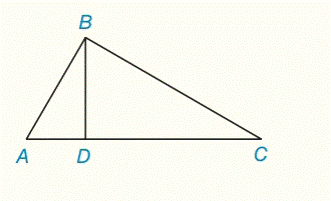Chapter 5.CR, Problem 31CR### Elementary Geometry for College St...

6th Edition
Daniel C. Alexander + 1 other
ISBN: 9781285195698

#### Solutions

Chapter
Section### Elementary Geometry for College St...

6th Edition
Daniel C. Alexander + 1 other
ISBN: 9781285195698
Textbook Problem
5 views

# Given: Δ A B C       with     right     ∠ B A C A D ¯ ⊥ B C ¯ a ) B D = 12 , A D = 9 , D C = ? b ) D C = 5 , B C = 15 , A D = ? c ) A D = 2 , D C = 8 , A B = ? d ) A B = 2 6 , D C = 2 , D C = ?To determine

a)

To find:

DC

Explanation

Given:

And

That is,

Approach:

By using angle-bisector theorem, that is “if a ray bisects of an angle of a triangle, then it divides the opposite side into two segments whose length are proportional to the lengths of the two sides that from the bisected angle”

Let x be the length of DC

To determine

b)

To find:

To determine

c)

To find:

AB

To determine

d)

To find:

### Still sussing out bartleby?

Check out a sample textbook solution.

See a sample solution

#### The Solution to Your Study Problems

Bartleby provides explanations to thousands of textbook problems written by our experts, many with advanced degrees!

Get Started

#### Domain Find the domain of the function. 20. k(x)=x+3x1

Precalculus: Mathematics for Calculus (Standalone Book)

#### Solve the equations in Exercises 126. 14x2=0

Finite Mathematics and Applied Calculus (MindTap Course List)

#### Squeeze Theorem In your own words, explain the Squeeze Theorem.

Calculus: Early Transcendental Functions (MindTap Course List)

#### For y = x3 ln x, y = a) x2(1 + 3 ln x) b) x3 + 3x2 (ln x) c) 3x d) 4x2(ln x)

Study Guide for Stewart's Single Variable Calculus: Early Transcendentals, 8th